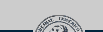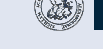# Pattern Recognition [PR]

Summary
This lecture gives an introduction into the basic and commonly used classification concepts. First the necessary statistical concepts are revised and the Bayes classifier is introduced. Further concepts include generative and discriminative models such as the Gaussian classifier and Naive Bayes, and logistic regression, Linear Discriminant Analysis, the Perceptron and Support Vector Machines (SVMs). Finally more complex methods like the Expectation Maximization Algorithm, which is used to estimate the parameters of Gaussian Mixture Models (GMM), are discussed. In addition to the mentioned classifiers, methods necessary for practical application like dimensionality reduction, optimization methods and the use of kernel functions are explained. Finally, we focus on Independent Component Analysis (ICA), combine weak classifiers to get a strong one (AdaBoost), and discuss the performance of machine classifiers. In the tutorials the methods and procedures that are presented in this lecture are illustrated using theoretical and practical exercises.

Dates & Rooms:
Wednesday, 9:00 - 9:45; Room: H4
Tuesday, 8:30 - 10:00; Room: H10

Lecturer
Steidl, Stefan

Overview

Exercises